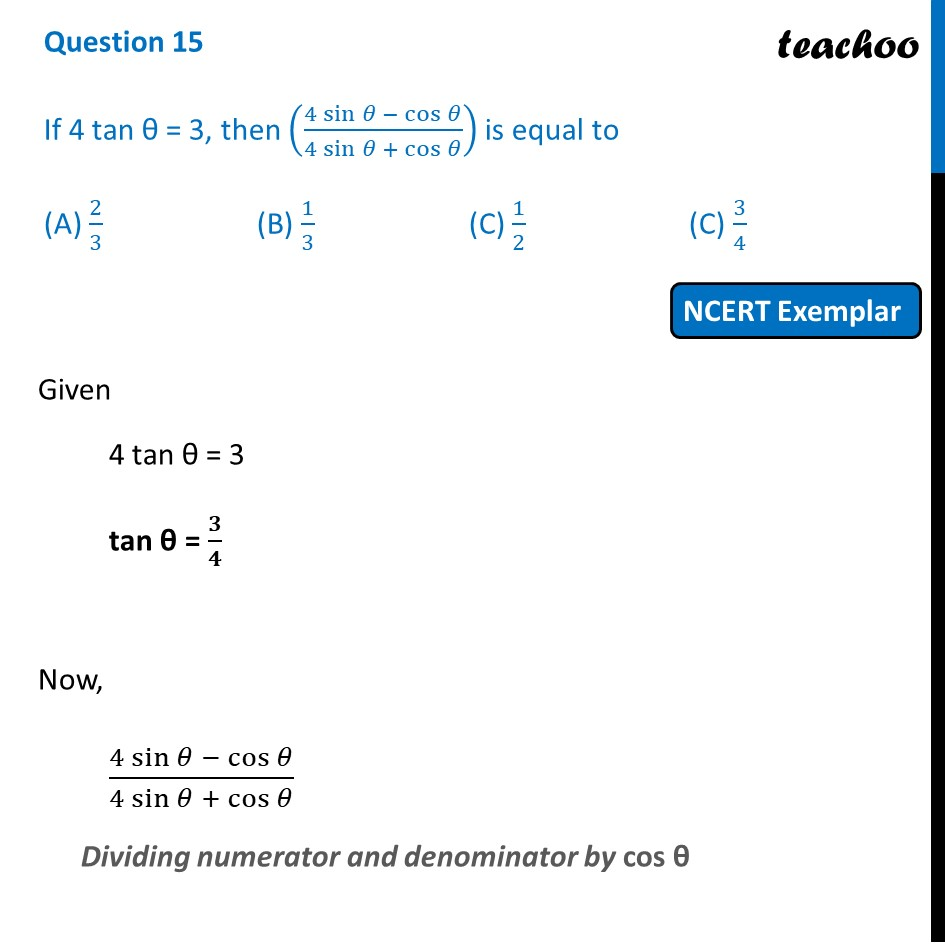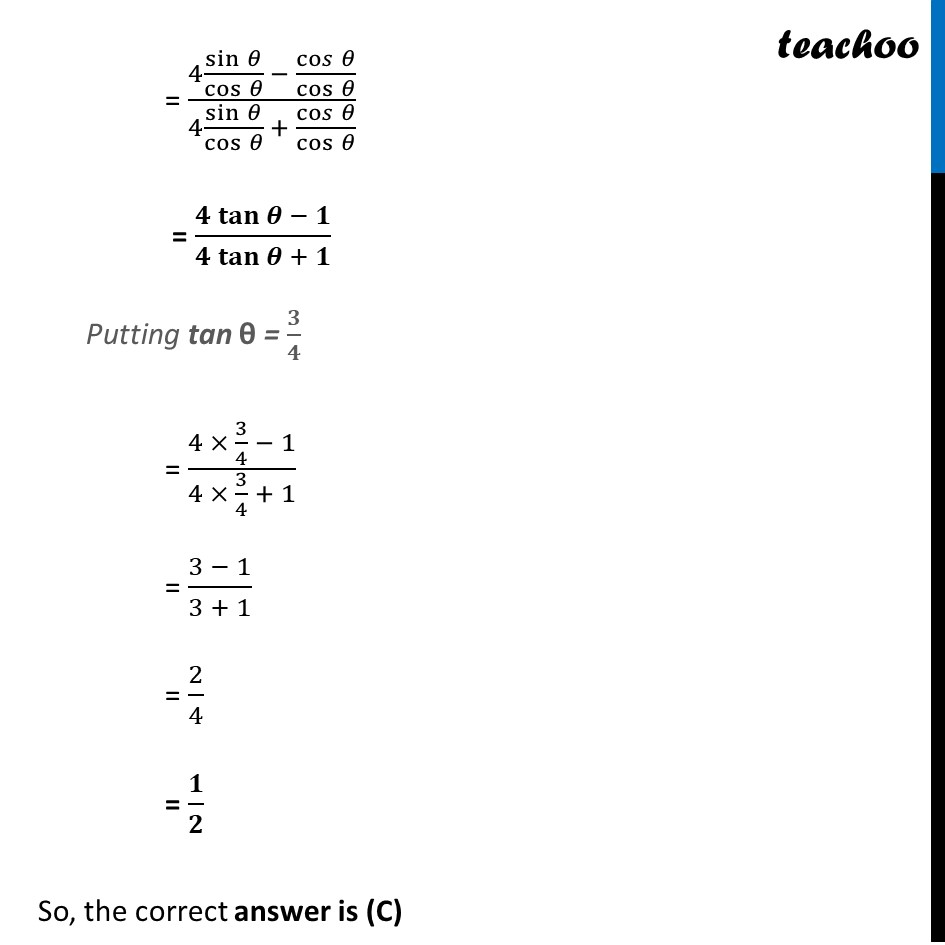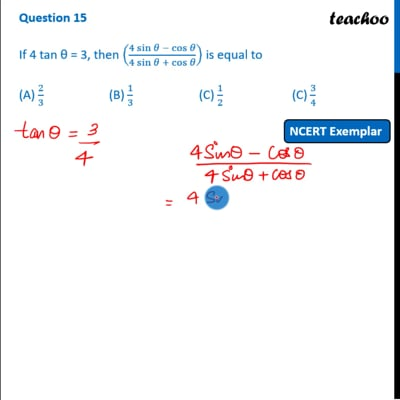NCERT Exemplar - MCQ

Chapter 8 Class 10 Introduction to Trignometry
Serial order wise

## (A) 2/3   (B) 1/3    (C) 1/2  (C) 3/4This video is only available for Teachoo black users

Introducing your new favourite teacher - Teachoo Black, at only ₹83 per month

### Transcript

Question 15 If 4 tan θ = 3, then ((4 sin⁡〖𝜃 − cos⁡𝜃 〗)/(4 sin⁡〖𝜃 + cos⁡𝜃 〗 )) is equal to (A) 2/3 (B) 1/3 (C) 1/2 (C) 3/4 Given 4 tan θ = 3 tan θ = 𝟑/𝟒 Now, (4 sin⁡〖𝜃 − cos⁡𝜃 〗)/(4 sin⁡〖𝜃 + cos⁡𝜃 〗 ) Dividing numerator and denominator by cos θ = (4 sin⁡𝜃/cos⁡𝜃 − co𝑠⁡𝜃/cos⁡𝜃 )/(4 sin⁡𝜃/cos⁡𝜃 + co𝑠⁡𝜃/cos⁡𝜃 ) = (𝟒 𝐭𝐚𝐧⁡〖𝜽 − 𝟏〗)/(𝟒 𝐭𝐚𝐧⁡〖𝜽 + 𝟏〗 ) Putting tan θ = 𝟑/𝟒 = (4 × 3/4 − 1)/(4 × 3/4 + 1) = (3 − 1)/(3 + 1) = 2/4 = 𝟏/𝟐 So, the correct answer is (C)